# Distortion Fields in OpenSCAD: Computationally Expensive And Not Entirely Satisfying, But Good To Know Anyway

Dec 10 2017 12:09pm

This is the second in an occasional series about 3D models and prints that I design. The code for this post, and some 3D models, are available in the associated github repo. The code for this post was largely inspired by the bending procedures posted to Thingiverse by the user flavius

In the [previous post]({{<ref "some_functions_for_vases.md">}}), I described how to use the `children` module in OpenSCAD to repeat models in regular patterns--specifically, to make cylindrical tiled vase sculptures. This post will explore what I call distortion fields--modules that apply non-homogenous transformations to their children. A word of warning: these techniques are extremely computationally expensive if you want good results in many common cases. They also tend to require some massaging to fit each specific case, so it's worth understanding how they work. The good news is that with a few helper functions, distortion fields are fairly simple to implement, so it's mostly a matter of coding for 10 minutes, pressing "render," and going to get a beer.

A good example of where a distortion field can be useful is in a model of an airplane wing (warning: I know nothing about actual aerodynamics). Wikipedia gives me a nice cross-section of an airfoil.I'd like to take that cross-section and use `linear_extrude` to stretch it out into a wing.

``````linear_extrude(height = 203) import("../dxf/airfoil.dxf");
``````But that's not right. Wings are wider at their base, where they connect to the fuselage, and narrower at their tip. They're also usually more triangular, in top-down view, than rectangular. What I need to make this extruded airfoil into a wing is to apply a kind of "scale-gradient," so that it gets smaller from one end to the other, and also a "translation-gradient" so that it sweeps back from the big end to the small end.

The `linear_extrude` function does have a `scale` parameter, which will help a little bit in this case.

``````module scaled_extruded_airfoil() {
linear_extrude(height = 203, scale=[0.3, 0.3]) {
import("../dxf/airfoil.dxf");
}
}

scaled_extruded_airfoil();
``````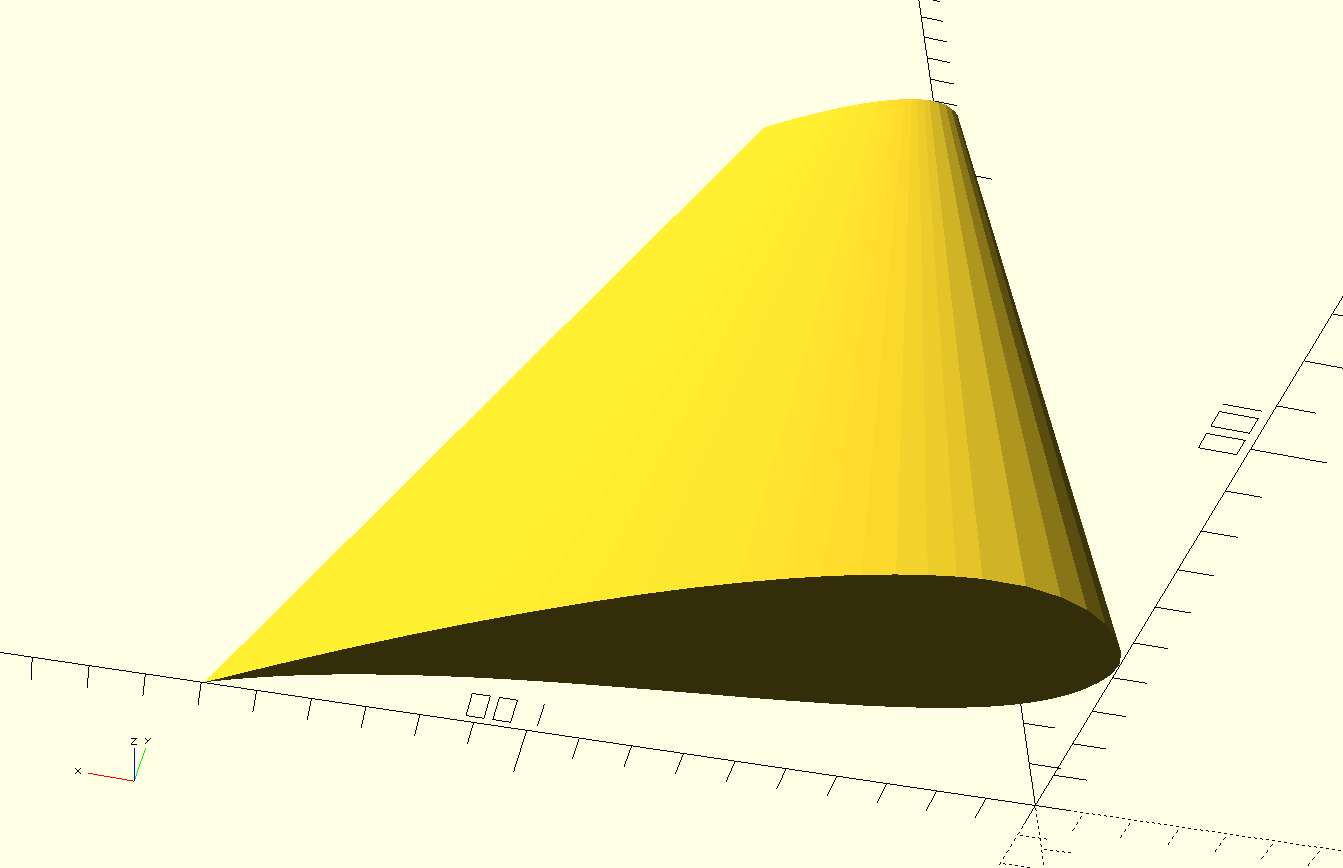This is good, but it doesn't give you a way to control where the end of the extruded shape "lands." The leading edge of the wing is a straight line, based on some internal representation of the origin of the 2D shape being extruded. I'd like the leading edge of the wing to be swept back. Also, in many cases the model to be scaled will be 3D, so this nice `linear_extrude` scaling trick won't be available. Or I might want to scale an object in a way that is not linear along its length, and this trick won't be suitable.

One way to try to achieve a "scaled and swept-back" effect is with a clever use of `hull` to unite a big, thin airfoil cross-section with a smaller cross-section translated one wing-length away. This is a good approach when it works, because it is far cheaper, and the result far smoother, than using a distortion field, as we'll see. But `hull` has its own price: it eliminates any concave details in the model. Holes and indentations, like the scooped bottom of the airfoil, do not survive.

``````module hull_wing() {
hull() {
linear_extrude(height = 0.5) {
scale([0.2, 0.2, 1]) import("../dxf/airfoil.dxf");
}
translate([-100, 0, 203]) {
linear_extrude(height = 0.5) import("../dxf/airfoil.dxf");
}
}
}

hull_wing();
``````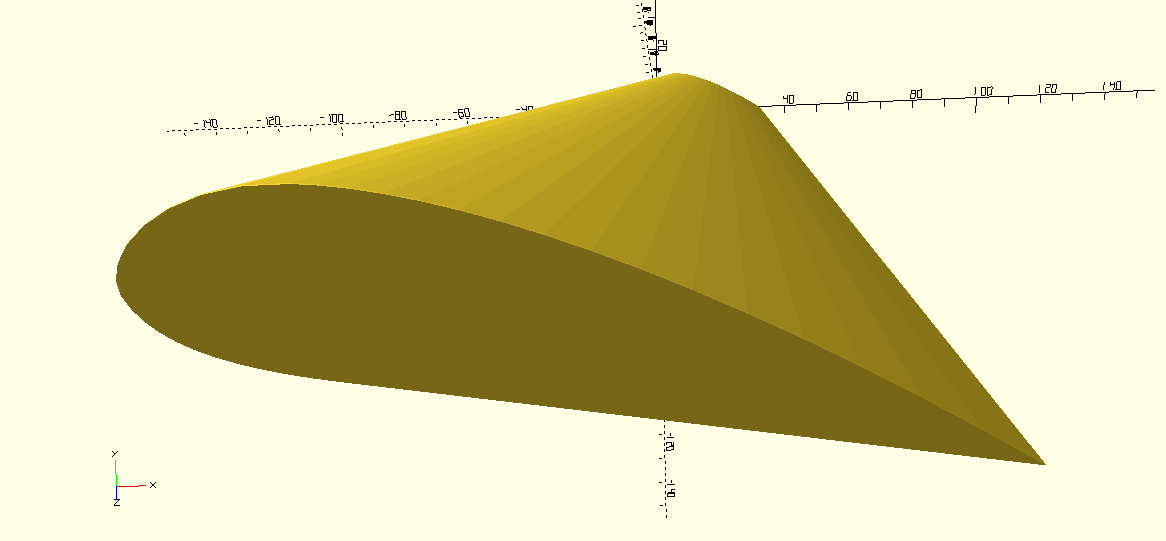As an introduction to how distortion fields work, let's look at how OpenSCAD models cylinders. If I make a simple cylinder, the result will be a prism with very obvious facets, not a smoothly-curved one.

``````cylinder(10, d-5);
``````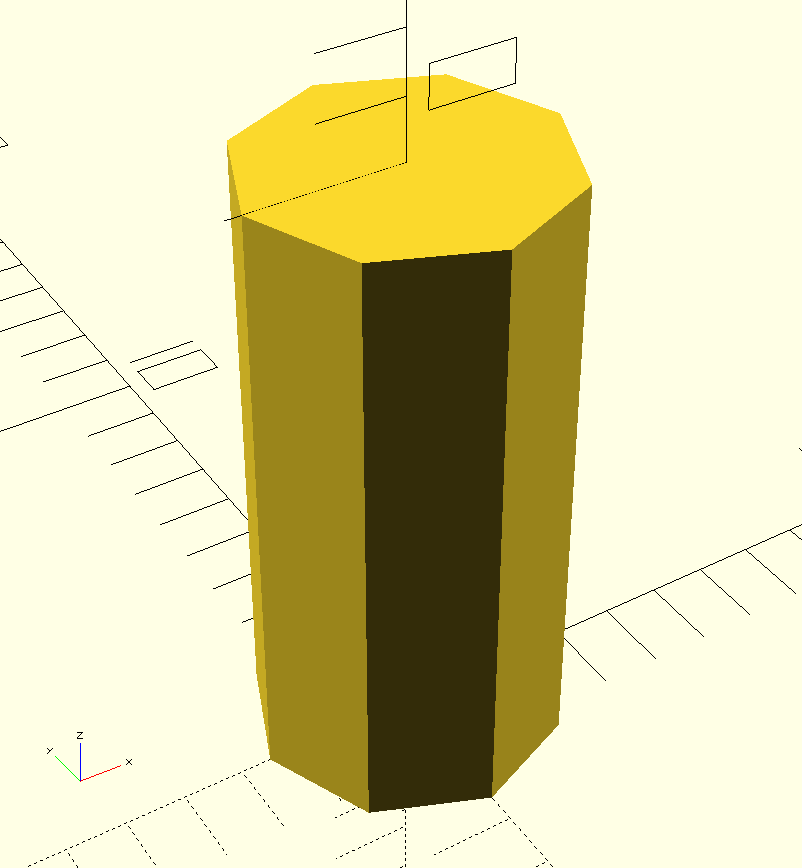If I want a cylinder with a smooth surface, I can manually specify the number of facets using the `\$fn` parameter.

``````cylinder(10, d-5, \$fn=30);
``````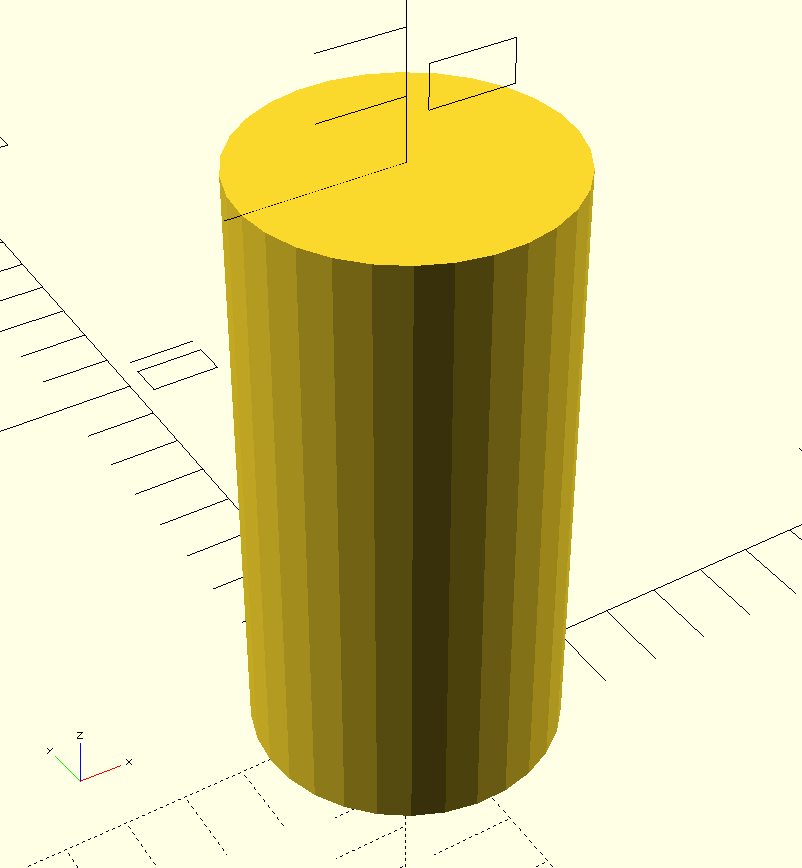The best number of facets is the lowest number that still looks like a curve in the eventual object. I'm usually making models for 3D printing, and I know that when each facet is small enough, the printer is just going to print a smooth curve. The central idea is that small-enough details blend together.

To apply that idea to the wing model, I can imagine applying successive transformations to arbitrarily-small slices of the wing, so that the final result would be a relatively-smooth approximation of the shape I wanted. I could make thin wing slices with successive calls to `linear_extrude,` but for the sake of demonstration I'm going to pretend that I only have a 3D model to work with, which is often the case in practice. I can take a short, wide cylinder (really any shape that can surround the 2D wing shape) and superimpose it on the wing.

``````module extruded_airfoil_cylinder_superimposed() {
linear_extrude(height = 203) import("../dxf/airfoil.dxf");
color("red") translate([100, 0, 0]) cylinder(1, d=300);
}

extruded_airfoil_cylinder_superimposed();
``````Now, if I take the intersection of these shapes, I should be left with a slice of the wing that has the same (thin) height as the red cylinder.

``````module intersection_airfoil_cylinder() {
intersection() {
linear_extrude(height = 203) import("../dxf/airfoil.dxf");
color("red") translate([100, 0, 0]) cylinder(1, d=300);
}
}

intersection_airfoil_cylinder();
``````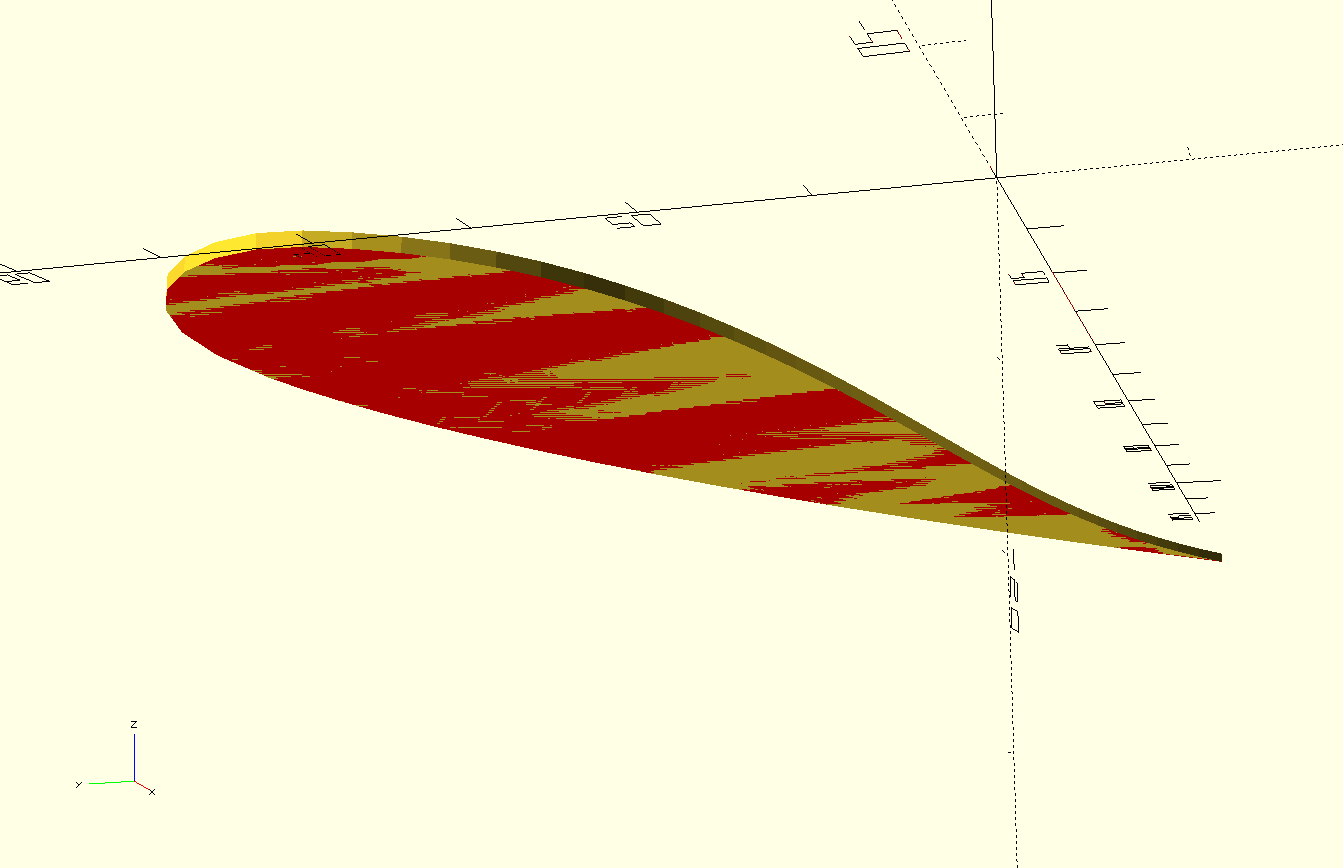There's a special kind of tipping point I always look for in any technical project. It's the point where I've figured out, not exactly how to do the thing I want, but enough to know that it can be done. Sometimes the process of getting to that tipping point is called "driving out risk," with the idea that once you hit it, there are no more risks that have the potential to fundamentally prevent what you want to do. After I know that I have all the building blocks to realize my idea, my next step is to get a working version, no matter mow ugly. Once I have a working version I can refine it as necessary (but it's surprising how often an initial version works for all the cases that matter!).

At this point I have all the building blocks I need to make the wing.

• I can make a thin slice of an object if I know its approximate dimensions in X and Y.
• I can scale and translate a thin slice of an object.
• I can use the `children` module to refer to an object to be distorted.

In pseudocode, the algorithm I want to implement looks like:

``````// This isn't real code!
// start and end are [x, y, z] vectors for the translation.
module translate_field(diameter, height, steps, start, end) {
for (i=[0:steps - 1]) {
// take the intersection of the transformed object and
// a cylinder to cut out a thin slice
intersection() {
// apply the appropriate transformation to the object.
// note that the interpolate function doesn't
//exist in OpenSCAD, I need to write it myself
translate(interpolate(start, end, steps, step)) {
children(0);
}
// translate a thin cylinder to the correct height for this
// step on the original object
translate([0, 0, i * (height / steps)]) {
cylinder((height / steps), d=diameter);
}
}
}
}
``````

Functions in OpenSCAD have a kind of odd syntax. I haven't looked into it in depth, but when I need to write one I often refer to the documentation. Suffice it to say that the `interpolate` function can be implemented this way:

``````function interp(start, end, steps, step) =
let (pct = step / steps)
[
start + (pct * (end - start)),
start + (pct * (end - start)),
start + (pct * (end - start)),
];
``````

This is a good linear interpolation function, but I actually don't want to permit translation in the Z-axis, so I can write a helper function that prevents it.

``````function xy_interp(start, end, steps, step) =
interp([start, start, 0], [end, end, 0], steps, step);
``````

Now I should be able to use this function to complete the `translate_field` function and use it to modify the scaled extruded airfoil.

``````// start and end are [x, y, z] vectors for the translation.
module translate_field(diameter, height, steps, start, end) {
for (i=[0:steps - 1]) {
// take the intersection of the transformed object and
// a cylinder to cut out a thin slice
intersection() {
// apply the appropriate transformation to the object.
// note that the interpolate function doesn't
//exist in OpenSCAD, I need to write it myself
translate(xy_interp(start, end, steps, i)) {
children(0);
}
// translate a thin cylinder to the correct height for this
// step on the original object
translate([0, 0, i * (height / steps)]) {
cylinder((height / steps), d=diameter);
}
}
}
}

module scaled_translated_wing(steps) {
translate_field(600, 203, steps, [0, 0, 0], [100, 0, 0]) {
scaled_extruded_airfoil();
}
}

translate([-200, 0, 0]) scaled_translated_wing(20);
scaled_translated_wing(50);
translate([200, 0, 0]) scaled_translated_wing(500);
``````

{{